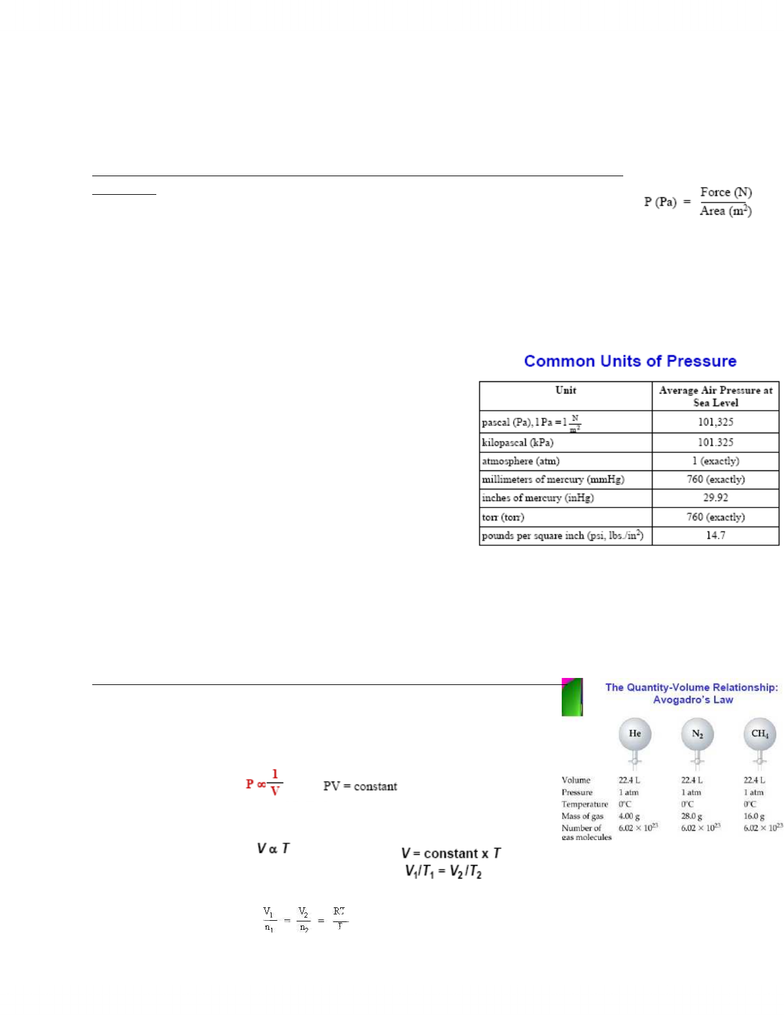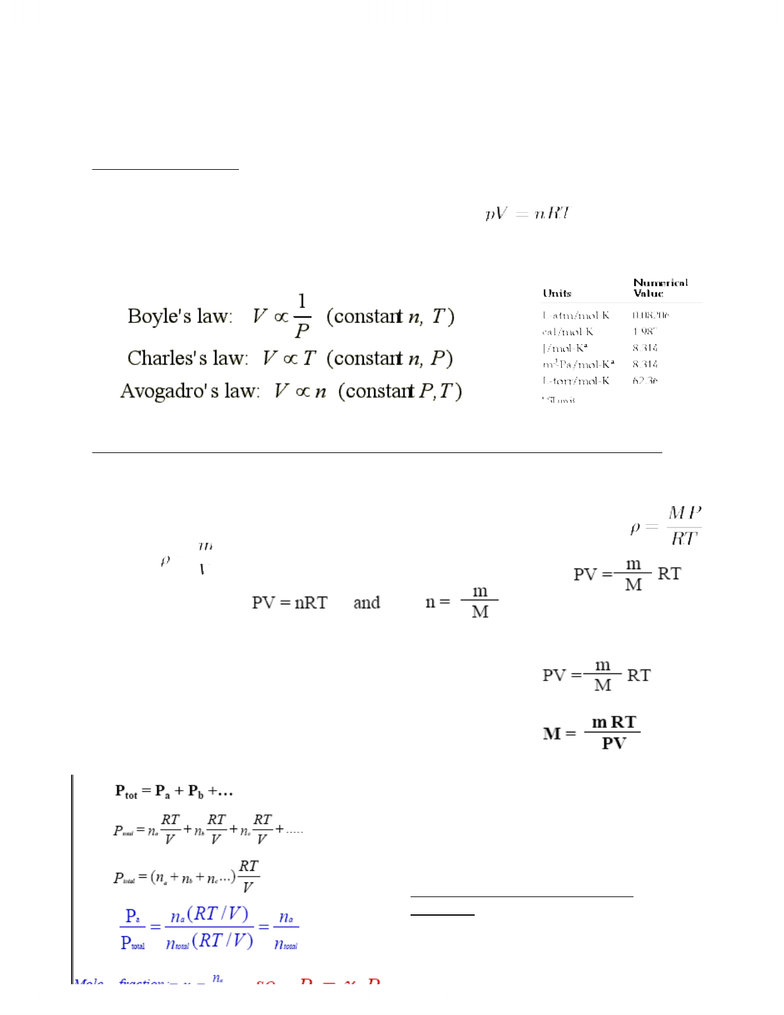# CHMA10H3 Chapter Notes - Chapter 5: Lead

33 views5 pagesChapter 5 Gases
5.1 Breathing: Putting Pressure to Work & 5.2 Pressure: The Result of Molecular
Collisions
- Pressure: Force exerted/ unit area, the sums of all molecular collisions/constant
force on surfaces to any gas
- Gases in the Air: Gas mixtures are always homogenous and are compressible
- Characteristics of Gases:
Gases assume volume and shape of containers
Gases are the most compressible state of
matter
Gases will mix evenly and completely when
confined in same container
Gas have lower densities than liquid or gases
- The pressure exerted by a solid: Both cylinders have
the same mass and have different areas of contact
- The pressure exerted by a liquid depends on height
and density of the column of liquid
- The pressure exerted by a gas depends on the # of gas particles in a given volume, the
volume of the contained and the average speed of the gas particles
5.3 The Simple Gas Laws: Boyles Law, Charles Law and Avogadros Law
- A SAMPLE OF GAS HAS 4 BASIC PHYSICAL PROPERTIES:
PRESSURE (P), VOLUME (V), TEMPERATURE (T) and AMOUNT OF MOLES (n)
1) BOYLES LAW: P1V1 = P2V2
2) CHARLES LAW: P & n = constant
www.notesolution.com
Unlock document

This preview shows pages 1-2 of the document.
Unlock all 5 pages and 3 million more documents.5.4 The Ideal Gas Law
- The equation state of a hypothetical ideal gas The state of an amount of gas is
determined by its pressure, volume, and temperature.
The modern form of the equation is: R =
5.5 Applications of the Ideal Gas Law: Molar Volume, Density, and Molar Mass of a Gas
- Molar Volume: Volume occupied by 1 mole of substance, usually under Standard
Temperature (T = 0 Degrees or 273K) and Pressure (P=1.00 atm) (STP),
is 22.4L
- Density: m= Molar Mass, V= molar
volume

- Molar Mass of a Gas:
5.6 Mixtures of Gases and Partial
Pressures
www.notesolution.com
Unlock document

This preview shows pages 1-2 of the document.
Unlock all 5 pages and 3 million more documents.